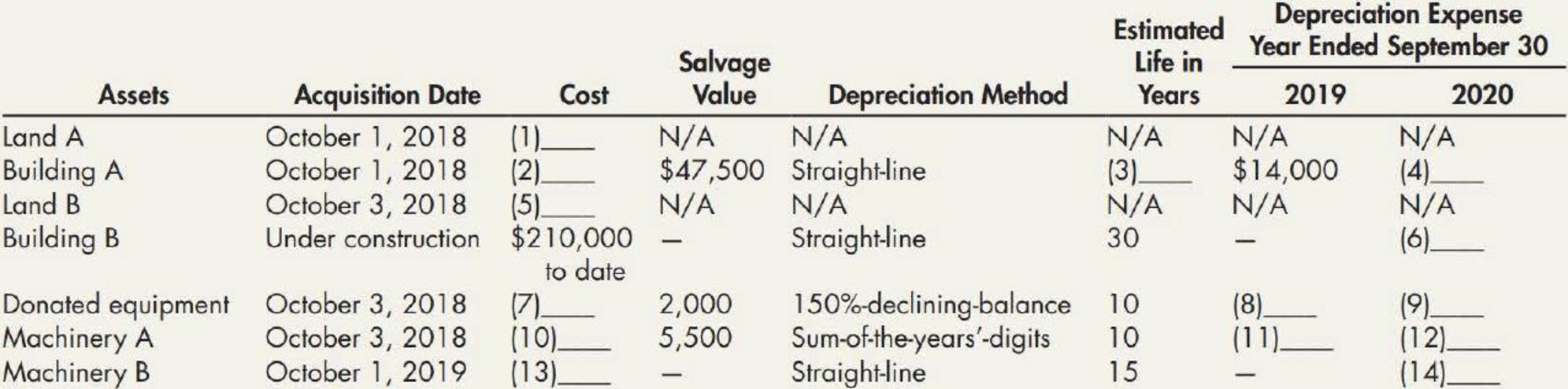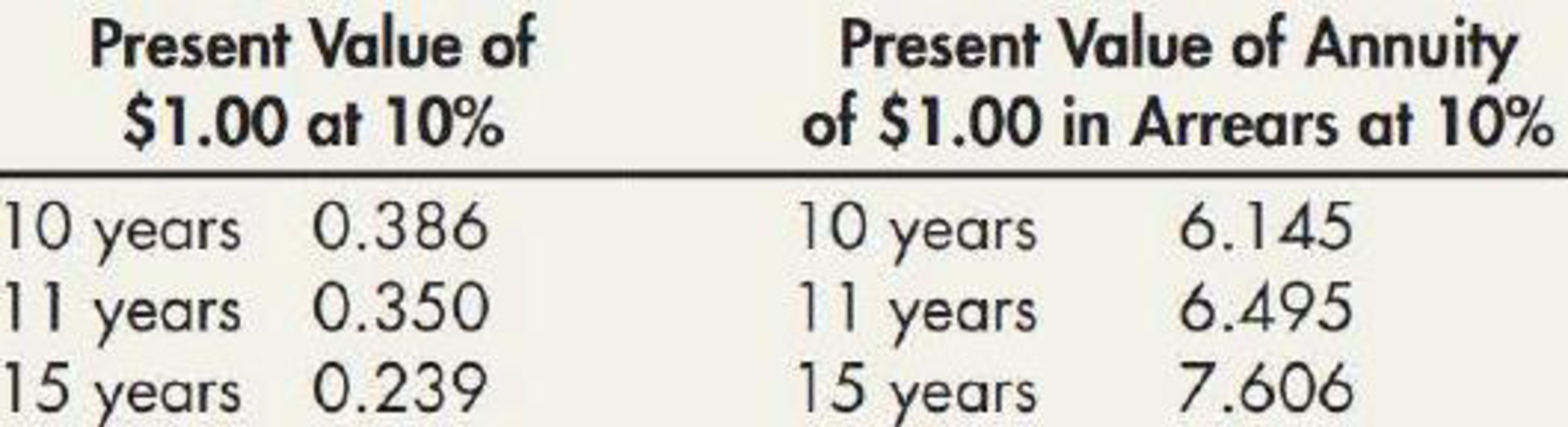Chapter 11, Problem 15P### Intermediate Accounting: Reporting...

3rd Edition
James M. Wahlen + 2 others
ISBN: 9781337788281

#### Solutions

Chapter
Section### Intermediate Accounting: Reporting...

3rd Edition
James M. Wahlen + 2 others
ISBN: 9781337788281
Textbook Problem
1 views

# Logan Corporation, a manufacturer of steel products, began operations on October 1, 2018.You have been asked to complete this schedule. In addition to determining that the data already on the schedule are correct, you have obtained the following information from Logan’s records and personnel: 1. Depreciation expense is computed from the first of the month of acquisition to the first of the month of disposition. 2. Land A and Building A were acquired together for a lump-sum price of $812,500. At the time of acquisition, the land had an appraised value of$72,000, and the building had an appraised value of $828,000. 3. Land B was acquired on October 3, 2018, in exchange for 3,000 newly issued shares of Logan’s common stock. At the date of acquisition, the stock had a par value of$5 per share and a fair value of $25 per share. During October 2018, Logan paid$10,400 to demolish an existing building on this land so that it could construct a new building. 4. Construction of Building B on the newly acquired land began on October 2, 2019. By September 30, 2020, Logan had paid $210,000 of the estimated total construction costs of$300,000. Estimated completion and occupancy are July 2021. 5. Certain equipment was donated to the corporation by a local university. An independent appraisal of the equipment when donated placed the fair value at $16,000 and the salvage at$2,000. 6. Machinery A’s total cost of $110,000 includes installation expense of$550 and normal repairs and maintenance of $11,000. Salvage value is estimated at$5,500. Machinery A was sold on February 1, 2020. 7. On October 1, 2019, Machinery B was acquired with a down payment of $4,000 and the remaining payments to be made in 10 annual installments of$4,000 each beginning October 1, 2020. The prevailing interest rate was 10%. The data that follow were abstracted from present value tables:Required:For each numbered blank in the schedule, supply the correct amount. Round each answer to the nearest dollar. Show supporting computations in good form.

To determine

Calculate the missing amount of Company L.

Explanation

Depreciation expense: Depreciation expense is a non-cash expense, which is recorded on the income statement reflecting the consumption of economic benefits of long-term asset on account of its wear and tear or obsolesces.

Straight-line depreciation method: The depreciation method which assumes that the consumption of economic benefits of long-term asset could be distributed equally throughout the useful life of the asset is referred to as straight-line method.

Sum-of- the-years’ digits method: Sum-of-the years’ digits method determines the depreciation by multiplying the depreciable base and declining fraction.

Double-declining-balance method: The depreciation method which assumes that the consumption of economic benefits of long-term asset is high in the early years but gradually declines towards the end of its useful life, is referred to as double-declining-balance method.

Calculate the missing amount of Company L as follows:

Figure (1)

Working note (1):

Step 1: Calculate the proportion of the Land A and Building A.

 Particulars Appraisal value (A) Total appraisal value (B) Proportion (AB×100) Land A $72,000$900,000 8.00% Building A $828,000 9,00,000 92.00% Total$900,000 100.00%

Step 2: Calculate the cost of land A.

Cost of the land A = (Lum-sum price ×Proportion of the land A)=$812,500×8100=$65,000

Working note (2):

Calculate the cost of Building A.

Cost of the Building A = (Lum-sum price ×Proportion of the Building A)=$812,500×92100=$747,500

Working note (3):

Calculate the estimated useful life of building A.

Estimated useful life of Building A} = Cost of acquisation Residual valueAnnual depreciation expense=$747,500(2)$47,500$14,000=50 years Working note (4): Calculate the depreciation expense of Building A for 2020. In this case, company L uses straight line method for building A, and hence it would have same depreciation expense for its all useful life. So, the depreciation expense for 2020 is$14,000 (given) as same as the prior year (2019).

Working note (5):

Calculate the cost of Land B.

Cost of land B = [Number of share issued ×(Par value per share + Fair value per share)]+Cost of demolishing=[3,000 shares×($5 per share +$25 per share)]+$10,400=$75,000+$10,400=$85,400

Working note (6):

In this case, there is no depreciation expense of Building B for 2020, because building is not yet constructed fully (under construction).

Working note (7):

The fair value donated equipment is considered as the cost of equipment. Hence, the cost of donated equipment is $16,000 (given). Working note (8): Step 1: Compute the straight line rate. Useful life = 10 years Depreciation rate=100%10 years×1.5=15% Step 2: Calculate the depreciation expense of donated equipment for 2019. Depreciation expense=Aquisition cost of equipment×Depreciation Rate=$16,000 (7)×15100=\$2,400

Working note (9):

Calculate the depreciation expense of donated equipment for 2020

### Still sussing out bartleby?

Check out a sample textbook solution.

See a sample solution

#### The Solution to Your Study Problems

Bartleby provides explanations to thousands of textbook problems written by our experts, many with advanced degrees!

Get Started

#### What is inflation and what causes it?

Principles of Macroeconomics (MindTap Course List)

#### What is job analysis?

Foundations of Business (MindTap Course List)

#### TIME FOR A LUMP SUM TO DOUBLE How long will it take 200 to double if it cams the following rates? Compounding o...

Fundamentals of Financial Management, Concise Edition (with Thomson ONE - Business School Edition, 1 term (6 months) Printed Access Card) (MindTap Course List)